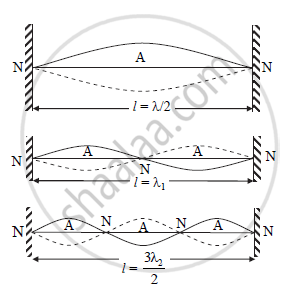# Show that all harmonics are present on a stretched string between two rigid supports - Physics

Show that all harmonics are present on a stretched string between two rigid supports.

#### SolutionVibration of string for different mode

Modes of vibrations in stretched string:-

a. Consider a string stretched between two rigid supports and plucked. Due to plucking, string vibrates and loops are formed in the string. Vibrations of string are as shown in figure.

b. Let,

p = number of loops

l = length of string

∴ Length of one loop = l /p                                                    ......(1)

c. Two successive nodes form a loop. Distance between two successive nodes is λ/2.

∴ Length of one loop = λ/2                                                     .......(2)

From equations (1) and (2),

λ/2 = l/p

∴ λ = 2l/p                                                                             ...........(3)

d. Velocity of transverse wave is given by,

v=sqrt(T/m)

e. Frequency of string is given by,

n = v/λ

Substituting λ from equation (3),

n=sqrt(T/m)/((2l)/p)

thereforen=p/(2l)sqrt(T/m)                                                                   .............(4)

f. Fundamental mode or first harmonic:-

In this case, p = 1

∴ From equation (4),

n=1/(2l)sqrt(T/m)

This frequency is called fundamental frequency.

g. First overtone or second harmonic:-

In this case, p = 2

∴ From equation (4),

n_1=2/(2l)sqrt(T/m)=2xx1/(2l)sqrt(T/m)=2n

thereforen_1=2n

h. Second overtone or third harmonic:-

In this case, p = 3

Using equation (4),

n_2=3/(2l)sqrt(T/m)=3xx1/(2l)sqrt(T/m)=3n

thereforen_2=3n

i. (p - 1)th overtone or pth harmonic:-

n_((p-1))=pxx1/(2l)sqrt(T/m)=pn

For pth overtone,

n_p=(p+1)/(2l)sqrt(T/m)=(p+1)n

j. Thus, in the vibration of stretched string, frequencies of vibrations are n, 2n, 3n,…..so on.

Hence, all harmonics (even as well as odd) are present in the vibrations of stretched string.

Concept: Study of Vibrations in a Finite Medium
Is there an error in this question or solution?
2014-2015 (October)

Share